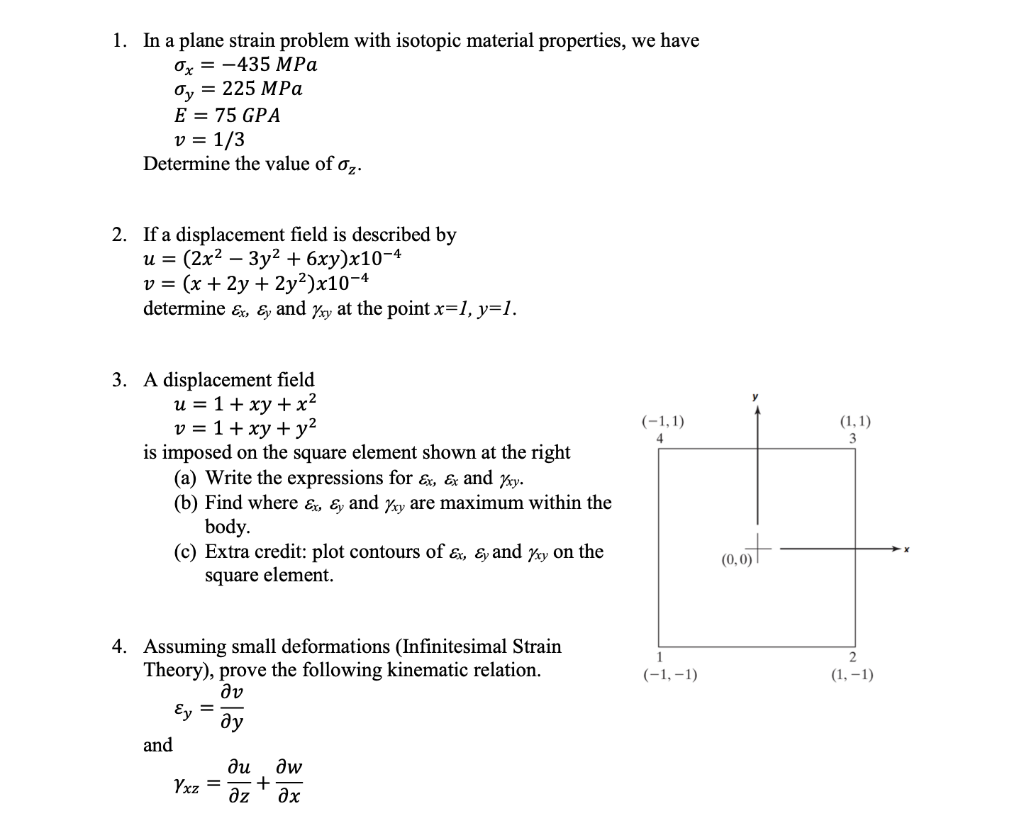Home / Answered Questions / Other / 1-in-a-plane-strain-problem-with-isotopic-material-properties-we-have-ox-435-mpa-oy-225-mpa-e-75-gpa-aw165

# (Solved): 1. In A Plane Strain Problem With Isotopic Material Properties, We Have Ox = -435 MPa Oy = 225 MPa E...1. In a plane strain problem with isotopic material properties, we have Ox = -435 MPa Oy = 225 MPa E = 75 GPA v = 1/3 Determine the value of oz. 2. If a displacement field is described by u= (2x2 â€“ 3y2 + 6xy)x10-4 v = (x + 2y + 2y2)x10-4 determine Ex, ey and Yxy at the point x=1, y=1. (-1,1) (1,1) 4 3. A displacement field u= 1 + xy + x2 v = 1 + xy + y2 is imposed on the square element shown at the right (a) Write the expressions for Ex, & and Yxy. (b) Find where Ex, Ey and yy are maximum within the body. (c) Extra credit: plot contours of ex, Ey and kry on the square element. (0,0) 4. Assuming small deformations (Infinitesimal Strain Theory), prove the following kinematic relation. av (-1,-1) (1, -1) Ey Oy and du Ow Yxz = Ã¶z tÉ™x

We have an Answer from Expert